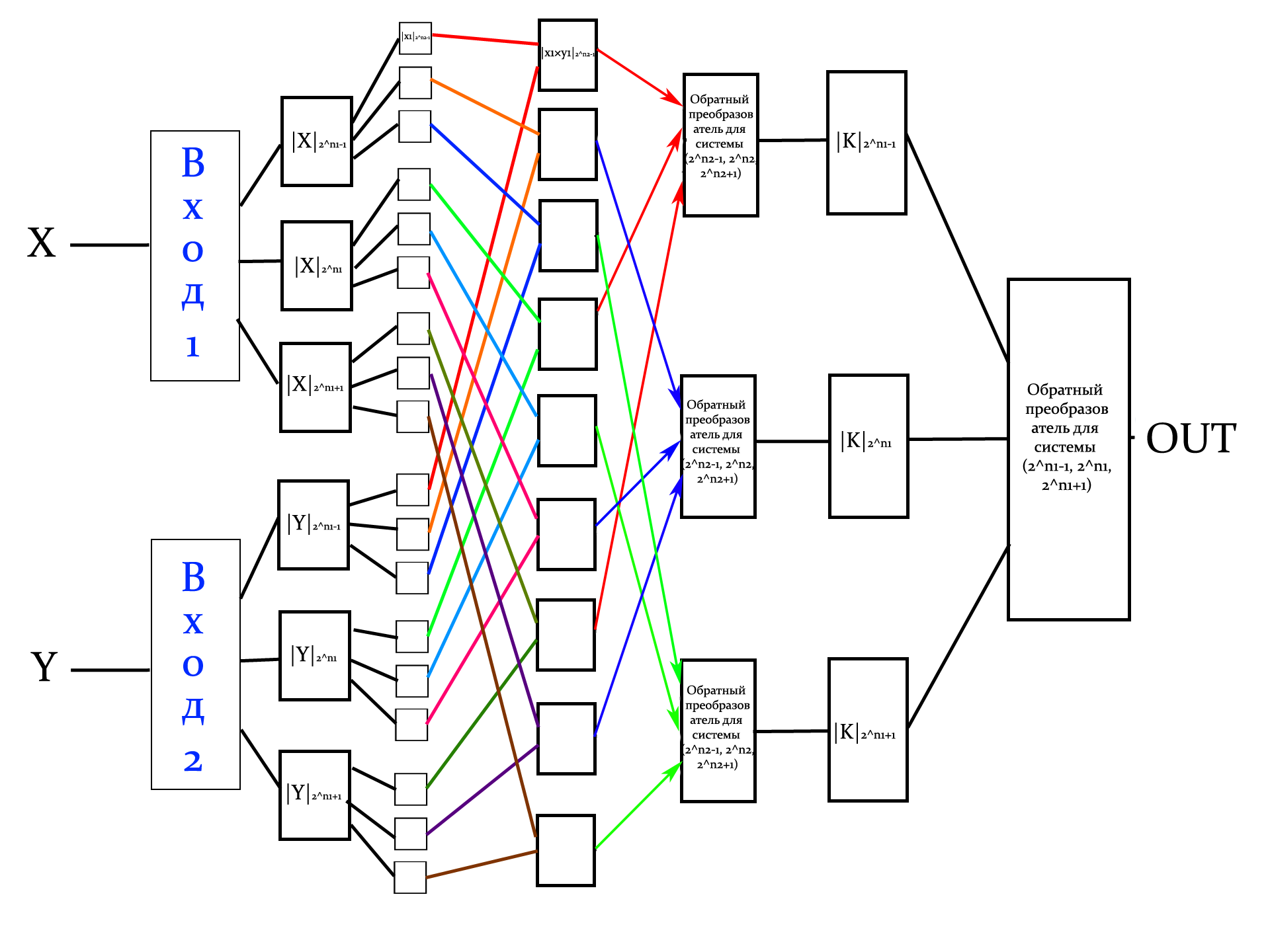# Verilog generator for multipliers based on recursive RNS arithmetic

Choose number of bits of multiplier inputs (from 5 to 64) :
Selected number of bits: 5 (Maximal output value: 1024)
Value of power n for basis 1: {2n-1, 2n, 2n+1}: 4 (Maximal value: 4080)
Value of power n1 for basis 2: {2n1-1, 2n1, 2n1+1}: 3 (Maximal value: 504 > (2n+1)2)
Basis 1: {24-1, 24, 24+1} or [15, 16, 17]
Basis 2: {23-1, 23, 23+1} or [7, 8, 9]

Description: Generator of conventional binary multiplier, where input operands have n bit length and output has 2*n bit length. Multiplication is done based on RNS arithmetic and special moduli sets {2n-1, 2n, 2n+1}, which are used twice in hierarchy. As the result, nine parallel channels are formed. However, two stages of direct and inverse converters are needed.

Work schemeFirst results (05.2013): For 32 bits this method is slower and takes more area than conventional RNS multiplication with basis {2n-1, 2n, 2n+1}. Delay is 6.87 compared to 4.30, area is 43401 compared to 31879. Possible variants of speed-up: merging of two levels of direct converters into one due to new mathematical tools, merging of reverse converters and intermediate module calculation. More research in this direction is needed.

Main page